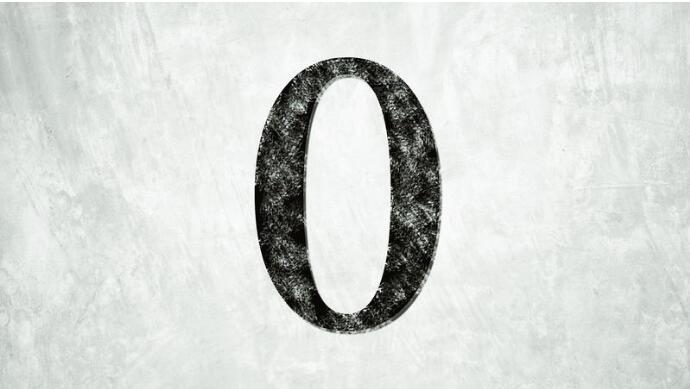When you were a little kid, even before you ever dealt with your first word problem in math class, you probably had to solve a problem something like this. You have four Starbursts, and you eat four Starbursts. What are you left with? That’s right: sadness. And also no candy. But though even small kids can understand “nothing,” the concept of “zero” is actually a bit more advanced; so advanced, in fact, that by the year 1200 C.E., it had only just barely reached the brightest mathematicians in Europe. This is the story of the invention of zero, and how a whole lot of nothing ended up changing the world.

## Making Something Out Of Nothing

It almost sounds impossible that ancient people wouldn’t have the concept of “zero.” Even animals can understand nothingness — just let your cat’s dish go empty if you don’t believe us. But there’s a big difference between nothing as a tangible emptiness and zero as a mathematical concept. One forerunner of the mathematical zero can be seen in the earliest known counting system, devised by the Sumerians. At first, they’d use a blank space to indicate a nothing value, and when that grew confusing, they began using a pair of angled wedges as a placeholder for a blank space. But in a sense, that symbol indicated a lack of a number, not a number in and of itself.

Similar placeholders for an empty value can be found in other counting systems, including those of the Mayans and the Babylonians. But most scholars agree that zero as a mathematical concept originated in India. The earliest use of the round symbol that would become the universal zero comes from the Bakhshali manuscript, a merchant’s document explaining mathematical equations for various transactions. It also included a placeholder zero in the form of a little black dot, and was in common parlance in India in the 3rd or 4th centuries C.E. Just a couple of centuries later, the symbol was used by legendary mathematical scholar Brahmagupta. In the 7th century, he wrote the earliest surviving explanation of how, exactly zero works: “When zero is added to a number or subtracted from a number, the number remains unchanged. A number multiplied by zero becomes zero.”

He also worked out that subtracting a positive number from zero gave you a negative number, and that subtracting a negative number from zero gave you a positive. That’s the first known account of knowing how zero works in relation to other numbers, and we can only assume he went on to coin the phrase, “Ditch the zero, get with the hero.”